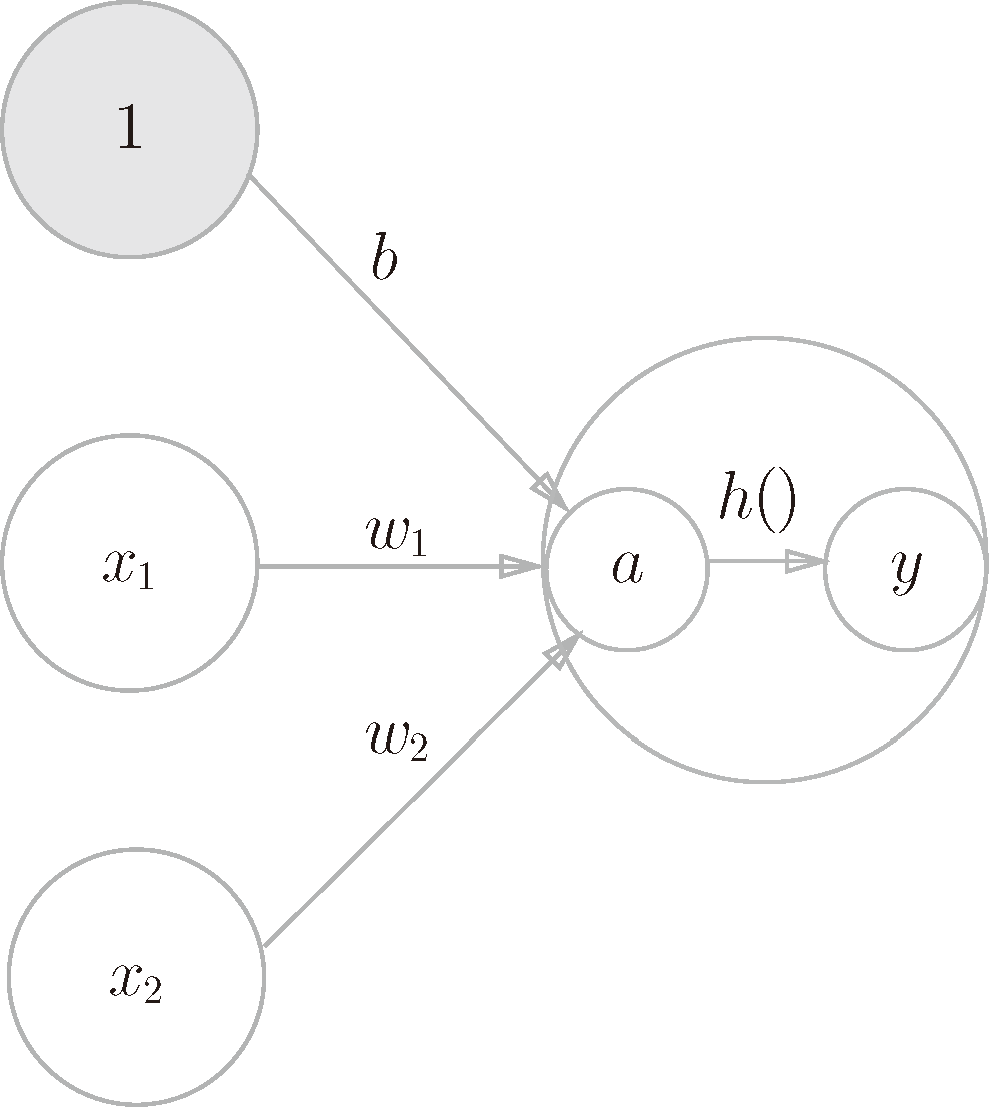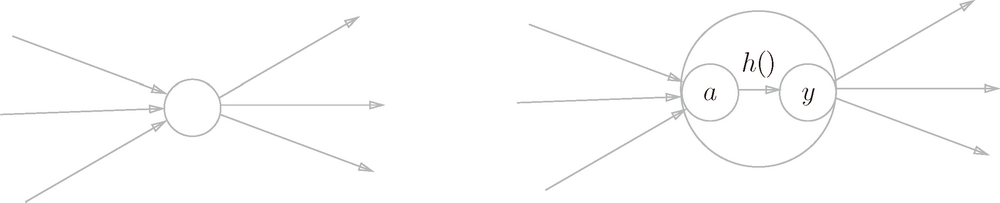# 深度学习入门：基于 Python 的理论与实现 (4)：神经网络 3.1.3(激活函数登场)

$a=b+w_1x_1+w_2x_2\quad\quad\quad\quad\quad(3.4)$

$y=h(a)\quad\quad\quad\quad\quad(3.5)$1 阶跃函数是指一旦输入超过阈值，就切换输出的函数。